# 初识Python之面向对象篇（中）

+关注继续查看
'''继承'''
'''定义并使用子类'''
class Car():
"""汽车之家"""
def __init__(self, manufacturer, model, year):#为实例化提供形参信息
self.manufacturer=manufacturer
self.model=model
self.year=year
def get_descriptive_name(self):#返回信息打印
long_name=str(self.year)+" "+self.manufacturer+" "+self.model
return long_name.title()
def update_obometer(self, mileage):#里程增加
else:
print("这是一个不合理的数据！")
def increament_odometer(self,miles):#智能加曲
class Bmw(Car):
'''这是一个子类，基类是Car'''
def __init__(self,manufacturer, model, year):
super().__init__(manufacturer, model, year)#这个是一个特殊的函数，它的功能是将父类和子类连接关联起来
# self.battery_size="6缸 3.0T"
self.Motor=Motor()#自动创建该motor实例，虽然没有指定参数，但是下面的函数指定了
# def motor(self):
#     print("发动机是"+str(self.battery_size))
class Motor(Bmw):
def __init__(self,Motor_size=60):
self.Motor_size=Motor_size
def describe_motor(self):
print("这款车的发动机参数是"+str(self.Motor_size)+"6缸，3.0T涡轮增压，225KW.")

my_tesla=Bmw("宝马","535Li","2017款")
print(my_tesla.get_descriptive_name())
my_tesla.update_obometer(0)
# my_tesla.motor()
print("*******************************")
my_tesla.increament_odometer(10)
my_tesla.Motor.describe_motor()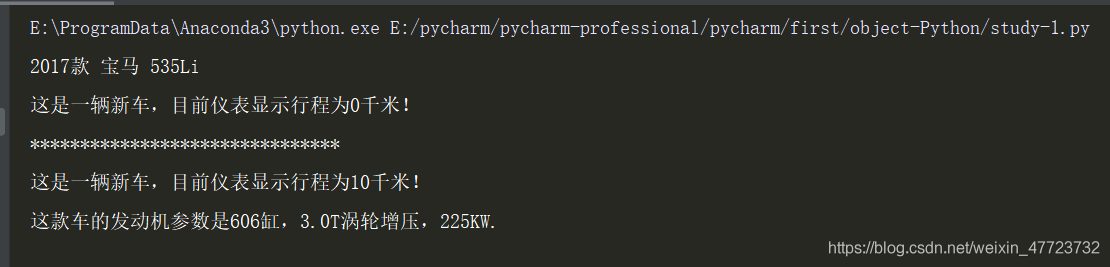'''多重继承'''
class Wai:
def __init__(self,x=0,y=0,color="black"):
self.x=x
self.y=y
self.color=color
def haijun(self,x,y):
self.x=x
self.y=y
print("发射鱼雷.......")
self.info()
def info(self):
print("定位目标：(%d,%d)"%(self.x,self.y))
def gongji(self):
print("导弹发射！！！")
class FlyWai(Wai):
def gongji(self):
print("飞船拦截！！！")
def fly(self,x,y):
print("火箭军........")
self.x=x
self.y=y
self.info()
flyWai=FlyWai(color="red")
flyWai.haijun(100,200)
flyWai.fly(12,15)
flyWai.gongji()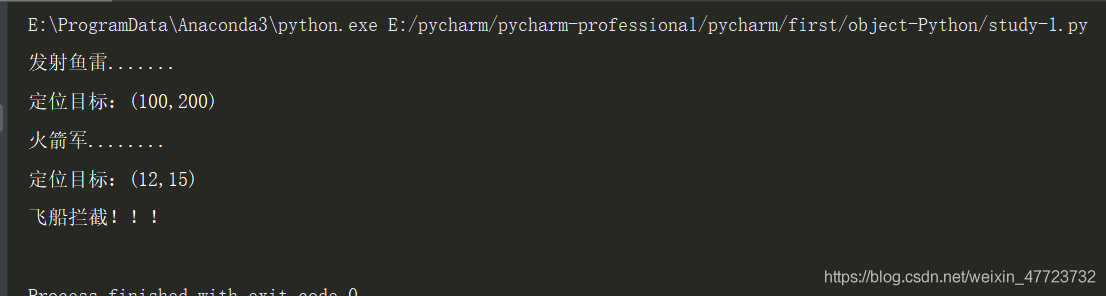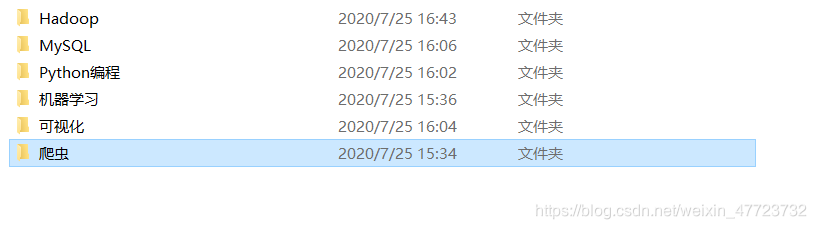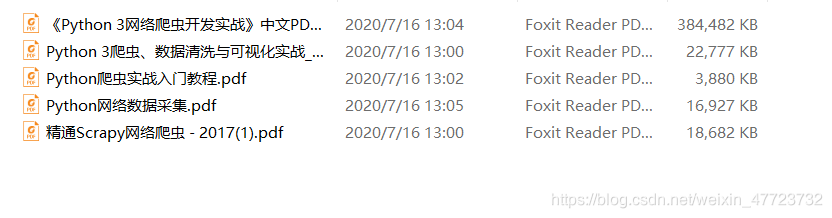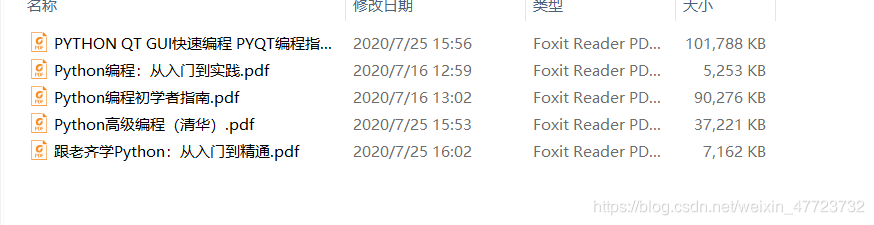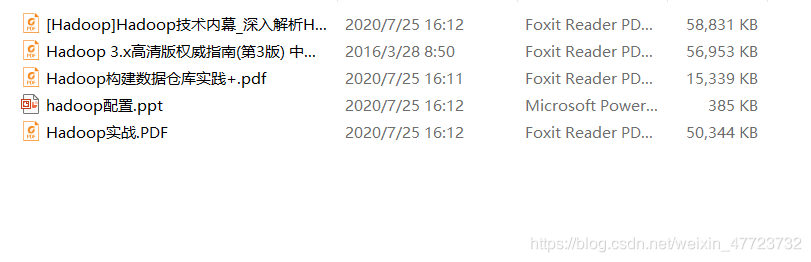## 满满的干货，都是小王在网络大数据的平台，细细挑选的，亲测试用，如果你不知道如何选择书籍，就快来领取福利吧！

'''模块架构'''
import math
from math import sqrt
import math as shuxue
a=math.sqrt(16)         #直接用模块加函数
b=sqrt(4)               #用函数
c=shuxue.sqrt(9)        #用自命名的模块加函数
print(a,"\t",b,"\t",c)

if __name__=="__main__":
print(a)
else:
print(c)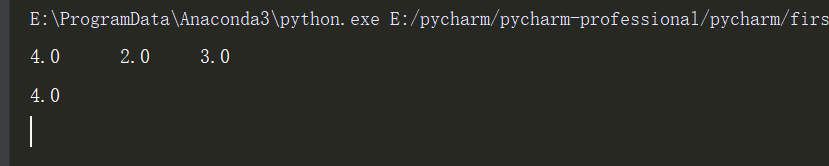"""使用包"""

'''例如上述实验方法，我们在导入包的时候，可以有不同的方法，因为导入不同，所以调用也不同

'''

'''导入类'''

'''类的导入与模块相似'''

'''迭代器'''
'''迭代器的使用例如for循环语句遍历迭代器'''

class Use:
def __init__(self,x=2,max=50):
self.__mul,self.__x=x,x     #初始化属性，X的初始值是2
self.__max=max
def __iter__(self):
return self
def __next__(self):
if self.__x and self.__x !=1:
self.__mul *=self.__max
if self.__mul <=self.__max:
return self.__mul
else:
raise StopIteration
if __name__=="__main__":
my=Use()
for i in my:
print("迭代数据元素为：",i)




'''使用内置迭代器方法iter（）'''
class Counter:
def __init__(self,x=0):
self.x=x
counter=Counter()
def used_iter():
counter.x+=2
return counter.x
for i in iter(used_iter,52):    #iter该函数用于函数，当最后一个参数迭代后返回和自己定义的相等的时候，程序结束，例如12
print(i)

from itertools import *
def height_class(h):
if h > 180:
return 'tall'
elif h < 180:
return "short"
else:
return "middle"
friends=[191,158,159,169,148,163,175,156,123,149,256,596,648,789,156,3589,180]
friends=sorted(friends,key=height_class)
for m,n in groupby(friends,key=height_class):#使用groupby（）遍历数据
print(m)
print(list(n))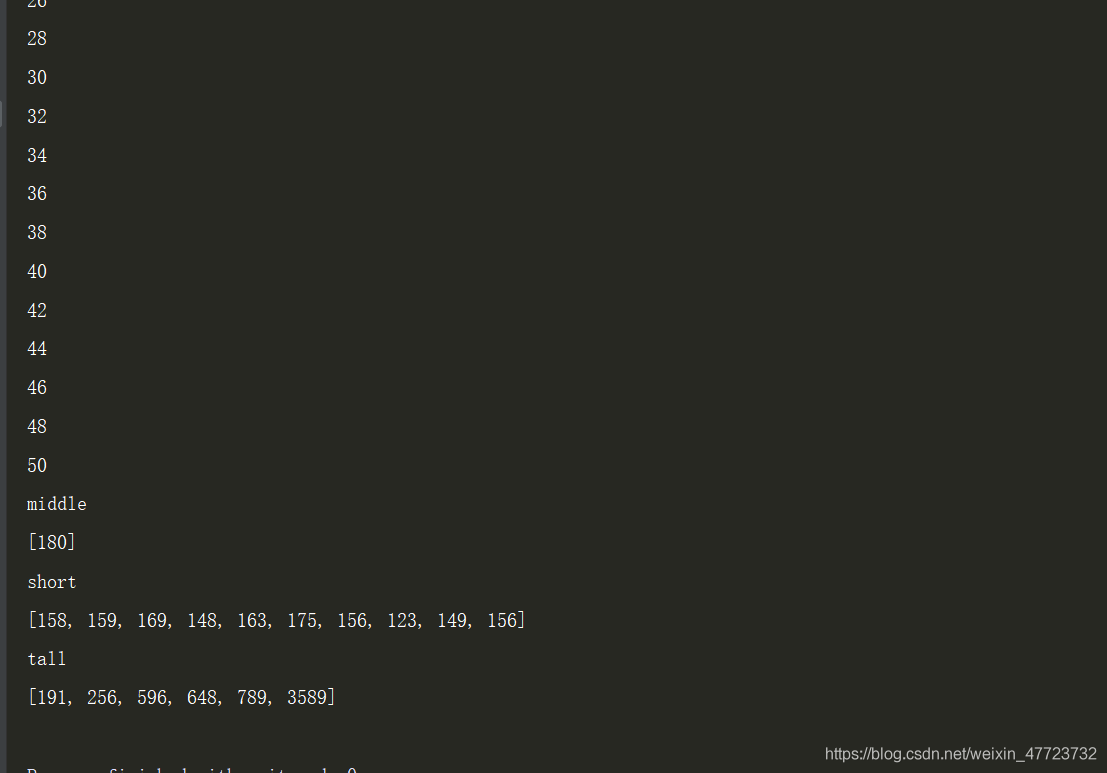Python-面向对象
Python-面向对象
20 0python面向对象之创建类
python面向对象之创建类
18 0Python面向对象

32 0【python】面向对象
1.简单写个例子，因为之前基本没写过python OOP。先写个父类 class Animal: # 构造方法 def __init__(self, name): self.
923 08.python之面相对象part.1(初识类与对象)
819 0python面向对象之类成员
1006 0python面向对象——方法
768 0Python面向对象之类的成员
877 0python面向对象
701 0723 0

268

0